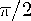Functions and CALL Routines

# ATAN Function

Returns the arc tangent.
 Category: Trigonometric

## Syntax

 ATAN (argument)

### Arguments

argument

specifies a numeric constant, variable, or expression.

The ATAN function returns the 2-quadrant arc tangent (inverse tangent) of the argument. The value that is returned is the angle (in radians) whose tangent is x and whose value ranges from -to. If the argument is missing, then ATAN returns a missing value.

The ATAN function is similar to the ATAN2 function except that ATAN2 calculates the arc tangent of the angle from the ratio of two arguments rather than from one argument.

SAS Statements Results
`x=atan(0);`
`0`
`x=atan(1);`
`0.7853981634`
`x=atan(-9.0);`
`-1.460139106`Previous Page | Next Page | Top of Page